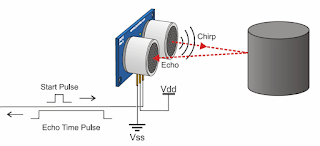# The Workings of the Ultrasonic Sensor## The workings of the ultrasonic sensor

In the ultrasonic sensor, an ultrasonic wave generated by a piezoelectric device called a certain frequency. This will produce a piezoelectric ultrasonic waves (generally frequency 40kHz) when an oscillator is applied to the object. In general, these tools will shoot the ultrasonic waves towards an area or target. After a wave touched the surface of the target, then the target will reflect the wave. Wave reflection from the target to be captured by the sensor, then the sensor calculates the difference between a transmitting and a reflected wave is received (Figure above).

Because the speed of sound is 340 m / s, then the formula for finding the distance by ultrasonic were:

where S is the distance between the ultrasonic sensor with an object (field reflection), and t is the time difference between the transmitting wave by the transmitter and the time when the reflected wave received by the receiver.

HC-SR04 ultrasonic sensor is ready for use, a tool that serves as a sender, receiver, and control of ultrasonic waves. This tool can be used to measure the distance of objects from 2cm - 4m with an accuracy of 3mm. Thus, for calculating the distance that only a maximum of 4 m, the above formula should be modified or customized units. The microcontroller can work on orders microseconds (μs 1s = 1,000,000) and units of distance we could change to a unit cm (1m = 100 cm). Therefore, the above formula can be updated to:

This tool has a 4 pin, pin Vcc, Gnd, Trigger, and Echo. Vcc pin for positive electricity and Gnd to ground her. Pin Trigger to trigger the release of the signal from the sensor and pin Echo to capture the signals reflected from the object.

How to use this tool are:

• When we leave a positive voltage on pin Trigger for 10μS, the sensor will send 8 step ultrasonic signal with a frequency of 40kHz
• Furthermore, the signal will be received at the pin Echo
• To measure the distance of objects that reflect these signals, the difference in time when the transmit and receive signals are used to determine the distance of the object
• - The formula for calculating the distance is S = (0.034 * t) / 2 cm.

Here is a visualization of the signal transmitted by the sensor HC-SR04